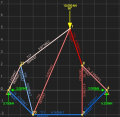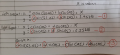# Truss Calculator problem

Joined Sep 28, 2021
196
Hi all,

below is a truss I simulated in a truss calculator. Just wondering, how to solve for the the compression and tension of each member and are there any method that can solve this? This is not homework, I just want to build a truss calculator app so I need to understand the physics behind it.

My solution is I consider the left and right support forces, however I only arrived at 3 equations with 4 unknows which cannot be solvedThank you for reading and have a nice day !!Last edited by a moderator:

#### MrAl

Joined Jun 17, 2014
8,992
Hi all,

below is a truss I simulated in a truss calculator. Just wondering, how to solve for the the compression and tension of each member and are there any method that can solve this? This is not homework, I just want to build a truss calculator app so I need to understand the physics behind it.

My solution is I consider the left and right support forces, however I only arrived at 3 equations with 4 unknows which cannot be solvedThank you for reading and have a nice day !!
View attachment 264708
View attachment 264709
Just saw this.

What you could do is start with just two branches. For example, have two ropes or chains holding up a given weight. Calculate the forces each rope has to endure. That shoudnt be too hard to do and you can search the web there will be a lot on this i believe. You can then generalize to more than two ropes or whatever.

#### hrs

Joined Jun 13, 2014
362
There are no ropes or chains MrAl, just trusses. If you can find a convenient entry point and the structure allows for it you can easily solve it by hand, one node at a time. If not you'll need to solve for 3 or more equations at a time some of the time.

Since you say you'd like to write an app I'd do it like this:
For each node set up one equation for the sum of forces in x and another for the sum of forces in y.
Write the system of equations as matrix.
Invert the matrix and multiply it with the load vector.

If you're still pursuing this, MrsssSu, I can write up an example after the weekend.

#### MrAl

Joined Jun 17, 2014
8,992
There are no ropes or chains MrAl, just trusses. If you can find a convenient entry point and the structure allows for it you can easily solve it by hand, one node at a time. If not you'll need to solve for 3 or more equations at a time some of the time.

Since you say you'd like to write an app I'd do it like this:
For each node set up one equation for the sum of forces in x and another for the sum of forces in y.
Write the system of equations as matrix.
Invert the matrix and multiply it with the load vector.

If you're still pursuing this, MrsssSu, I can write up an example after the weekend.
Hello,

Yes i know there are no ropes or chains i read the post. The point is, the forces will be similar although of course not in the same directions.
Check out some examples on the web.